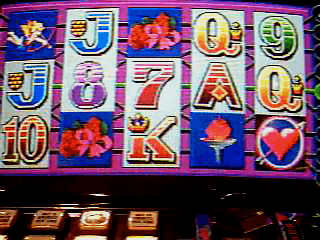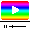# Sweet Hearts II

## Video Slot Machine published 28 years ago by Aristocrat Leisure Industries Pty

#### Listed in MAMEVideo (1)

Sweet Hearts II © 1994 Aristocrat.

Let Your Sweet HEART Win You Free Spins! A 5-reel video slot machine with a love theme.

TECHNICAL/MACHINE PICT.
1

Aristocrat MK2.5/MKIV hardware (1994)

MK2.5/MKIV hardware:
M6809 based.
304x216 screen resolution, 64 colours.

Artwork part numbers:
261705 - Belly Panel artwork, '(c) 1994 Aristocrat Leisure Industries' in a script font.
273937 - Belly Panel artwork, '(c) 1994 Aristocrat Technologies PTY LTD' in Helvetica at the bottom. Circa 2004.
275378 - Top Box Paytable (High-boy, MVP), '(c) 1994 Aristocrat Technologies PTY LTD' in Helvetica at the bottom. Circa 2004. Reel symbol graphics updated.
278952 - Top Box Paytable (Casino Top, MVP), '(c) 1994 Aristocrat Technologies PTY LTD' in Helvetica at the bottom. Circa 2004. Reel symbol graphics updated.

MKIV button layout:
Top row (90 credit version):
Collect
Bet 1 Credit
Bet 2 Credits
Bet 3 Credits
Bet 5 Credits
Bet 10 Credits

Bottom row:
Reserve
Play 1 Line / Red
Play 3 Lines
Play 5 Lines
Play 7 Lines
Play 9 Lines / Black
Gamble
Take Win

The Reserve button is replaced by a Service button on casino versions.

TRIVIA

Sweet Hearts II was released in September 1995.

On the artwork the title reads "Sweet Hearts II", but on the Lucky Devil version in the Electronic Game Information display, it says "Sweethearts II (Lucky Devil)". The 2000 MKV Hyperlink version was also called "Sweethearts II" on the artwork. The 2004 MKVI update reverts back to the original "Sweet Hearts II" in the game information display. Official title mess-ups?

Bet amounts differ between jurisdictions. For example, the 180 credit version has bet buttons marked 1, 2, 5, 10 and 20, whereas the 45 credit version's bet buttons are simply 1 through 5.

MKIV and MKV versions were removed from Victorian gaming venues, including Crown Casino due to new gaming machine laws. As of December 2007, no gaming machine is allowed to have an 'auto-spin' function, and machines must not accept \$100 notes.

On the MK2.5 and MKIV versions, the Seven through Ace symbols had a white or pale yellow background. On the later games all symbols have a blue reel background.

On the Jubilee MKIV version, the 'Heart' graphic had a black background with a circle around the heart. Later versions had a blue background with small hearts around it. Additionally, this version displays the total number of free spins played at the end of the feature. If 5 Hearts occur on this version, all five reels are held for the remaining free spins rather than being spun to their respective positions.

SCORING

All pays are left to right including scattered Hearts. Diamond substitutes for all symbols except Heart.

Paytable (standard version):

Diamond : 1 = 2, 2 = 25, 3 = 300, 4 = 2000, 5 = 5000
Flame : 1 = 1, 2 = 5, 3 = 120, 4 = 500, 5 = 2500
Drink : 1 = 1, 2 = 5, 3 = 120, 4 = 500, 5 = 2500
Roses : 2 = 5, 3 = 100, 4 = 250, 5 = 1000
Cupid : 2 = 5, 3 = 100, 4 = 250, 5 = 1000
Crown : 3 = 40, 4 = 75, 5 = 500
Ace : 3 = 40, 4 = 75, 5 = 500
King : 3 = 10, 4 = 50, 5 = 200
Queen : 3 = 10, 4 = 50, 5 = 200
Jack : 3 = 10, 4 = 40, 5 = 150
Ten : 3 = 10, 4 = 40, 5 = 150
Nine : 2 = 2, 3 = 5, 4 = 20, 5 = 120
Eight : 3 = 5, 4 = 20, 5 = 100
Seven : 3 = 5, 4 = 20, 5 = 100
Heart : 2 = 2, 3 = 5, 4 = 20, 5 = 50

Paytable (New Zealand version):

Diamond : 1 = 2, 2 = 25, 3 = 300, 4 = 750, 5 = 1500
Flame : 1 = 1, 2 = 5, 3 = 120, 4 = 500, 5 = 1000
Drink : 1 = 1, 2 = 5, 3 = 120, 4 = 500, 5 = 1000
Roses : 2 = 5, 3 = 100, 4 = 250, 5 = 500
Cupid : 2 = 5, 3 = 100, 4 = 250, 5 = 500
Crown : 3 = 40, 4 = 75, 5 = 500
Ace : 3 = 40, 4 = 75, 5 = 500
King : 3 = 10, 4 = 50, 5 = 200
Queen : 3 = 10, 4 = 50, 5 = 200
Jack : 3 = 10, 4 = 40, 5 = 150
Ten : 3 = 10, 4 = 40, 5 = 150
Nine : 2 = 2, 3 = 5, 4 = 20, 5 = 120
Eight : 3 = 5, 4 = 20, 5 = 100
Seven : 3 = 5, 4 = 20, 5 = 100
Heart : 2 = 2, 3 = 5, 4 = 20, 5 = 35

TIPS AND TRICKS

* All pays are left to right including scatters.

* Diamond substitutes for all symbols except scattered Heart.

* Feature : Any left to right scatter win triggers 3 free spins.
Initial scatter win is held during feature.
During feature scatters pay any.
Any additional Hearts spun up are held and 3 spins recommence.
When a 5-of-a-kind Heart win occurs the 5x Heart win is replayed 3 times.

* It is not possible to get a line win during the Heart spins due to the location of the symbols.

SERIES
SOURCES

Slot's picture.
Slot's ROM.